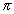IN MATH: 1. n. the ratio of a circle's circumference to its diameter (symbol is). EX. The area formula for a circle states:, area equals the product of pi and the square of the radius. EX. When written as a decimal,is nonterminating. One decimal expression foris 3.14159265358979323846264338327950288419 71693993751058209749445923078164062...

Circle
Circles are describe in terms of their radius or diameter.
The circumference is the perimeter of a circle.The circumference,
in terms of radius,or
in terms of diameter,The area,
in terms of radius,or
in terms of diameter,Using a radius of:      The ( or ) is:     square units or units.
 Using a diameter of:      The ( or ) is:     square units or units.

IN ENGLISH: 1. as defined above.

APPLICATION: See list 140.

1. State the formula for the volume of a sphere.This is a page from the dictionary MATH SPOKEN HERE!, published in 1995 by MATHEMATICAL CONCEPTS, inc., ISBN: 0-9623593-5-1.   You are hereby granted permission to make ONE printed copy of this page and its picture(s) for your PERSONAL and not-for-profit use.